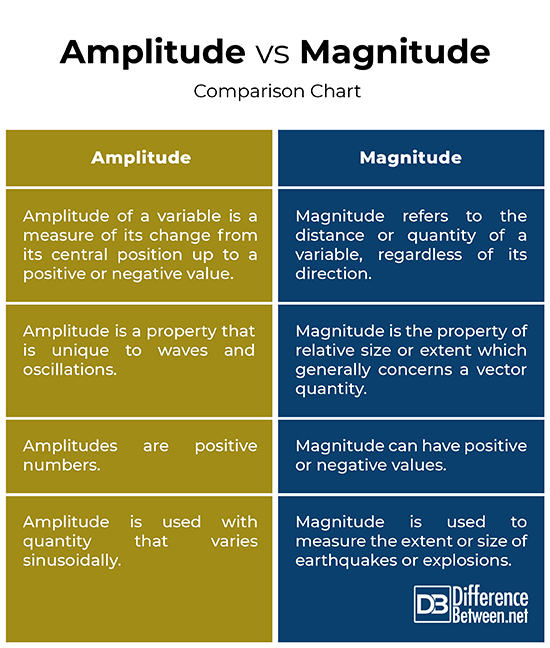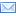#Difference Between Similar Terms and Objects

# Difference Between Magnitude and Amplitude

In layman terms, both the terms magnitude and amplitude are often used interchangeably to describe properties of quantities. When you check thesaurus, you find both the terms are synonymous. However, in engineering, they mean two different things and so it is important to understand the difference between the two terms. The amplitude of a variable is the measure of deviation of that variable from its central position up to a positive or negative value. It simply is the maximum displacement of a vibration or oscillation from its equilibrium position (zero level).  This is why signal amplitudes are either positive or negative values. The magnitude of a variable, on the other hand, is the measure of how far its quantity differs from zero, regardless of the direction. Thus, magnitudes are always positive numbers. We are here to discuss some key points comparing the two terms.## What is an Amplitude?

If you have ever put an object into the pond with still water, then you would know any disturbance in the water creates ripples or waves. Ever seen a wave in the ocean? It can be as little as a small ripple or big as a huge tsunami. Similarly, there are all kinds of waves around us that we experience every single day, such as electromagnetic waves, radio waves, or light waves, which we are unable to see or hear. But they are there. Water waves are in the visible form, though, but there are waves that we cannot see but they do exist. Amplitude simply is a measure of how big the wave is. Every wave has different amplitude, regardless of the kind. Waves can have same frequency and wavelength, but they can have different amplitudes. Amplitude refers to the maximum displacement of points on a wave measured from its central position.## What is a Magnitude?

Magnitude refers to the distance or quantity of a variable, regardless of its direction. In physics, the distance and the direction between two points can be represented by a quantity known as a vector. Magnitude is the length of the vector, while direction simply is a way from point A to point B. By contrast, quantities known as scalar quantities only have magnitude, so they can be described using just a real number. The magnitude of a scalar quantity is a scalar. Magnitude refers to the size of an object, which determines whether one object is larger or smaller than the other object. If you talk about magnitude of some object, you simply refer to its size or scale. For example, the magnitude of a football is greater than that of a tennis ball or a golf ball for that matter. Magnitude is used to measure the extent of an earthquake or an explosion.

## Difference between Magnitude and Amplitude

### Definition of Magnitude and Amplitude

– The amplitude of a variable is the measure of deviation of that variable from its central position up to a positive or negative value. It simply is the maximum displacement of a vibration or oscillation from its equilibrium position (zero level). Magnitude, on the other hand, refers to the distance or quantity of a variable, regardless of its direction. It refers to the size of an object, which determines whether one object is larger or smaller than the other object. Magnitude is a property more like area or volume, which doesn’t concern direction.

### Property of Magnitude and Amplitude

– Magnitude is the property of relative size or extent which generally concerns a vector quantity. When we are talking about magnitude of some object, we simply refer to its size or scale. Magnitude is a scalar quantity that possesses the size only, not direction. On the contrary, vector quantities have both magnitude and direction. Amplitude is a property that is unique to waves and oscillations. Amplitude is one of the most important physical characteristics of a wave, other being the wavelength. They are both measures of distance.

### Example

– Amplitude simply measures the length and width of waves from the resting position, such as sound waves, as they vibrate or move. The way radio waves or sound waves move back and forth and how much they move refers to its amplitude. Magnitude is used to measure the extent or size of an explosion or an earthquake. Vector quantities in physics are expressed with both magnitude and direction. For example, magnitude of movement is velocity. When we say 10m/s westward, it tells us both the magnitude of movement, which is 10 m/s, and the direction as well, which is westward.

### Amplitude vs. Magnitude: Comparison Chart## Summary of Magnitude vs. Amplitude

While both the terms are often used interchangeably, they are very different terms. Amplitude of a variable is simply a measure of change relative to its central position, whereas magnitude is a measure of distance or quantity of a variable irrespective of its direction. Amplitude is a property that is unique to waves and oscillations. Magnitude, on the other hand, is the property of relative size or extent which generally concerns a vector quantity. Amplitude is used with quantities that vary sinusoidally, while earthquakes are measured using magnitudes in Richter Scale.

Latest posts by Sagar Khillar (see all)

### Search DifferenceBetween.net :

Custom Search

Help us improve. Rate this post!(1 votes, average: 3.00 out of 5)Loading...Email This Post : If you like this article or our site. Please spread the word. Share it with your friends/family.

1. Dear Sagar, Thank you for your difference between narratives. They are very informative. I rated this one 1 star, perhaps too harsh, because the comparison chart is incorrect or inconsistent with the description of whether amplitude or magnitude can be a positive number. I believe it is stated correctly in the initial paragraph, but in the comparison chart is incorrectly stated. Hope this helps. I will still continue to read your content! Best, Eli

2. Assalam o alaikum
Great sir
Very important article .

Please note: comment moderation is enabled and may delay your comment. There is no need to resubmit your comment.

## References :